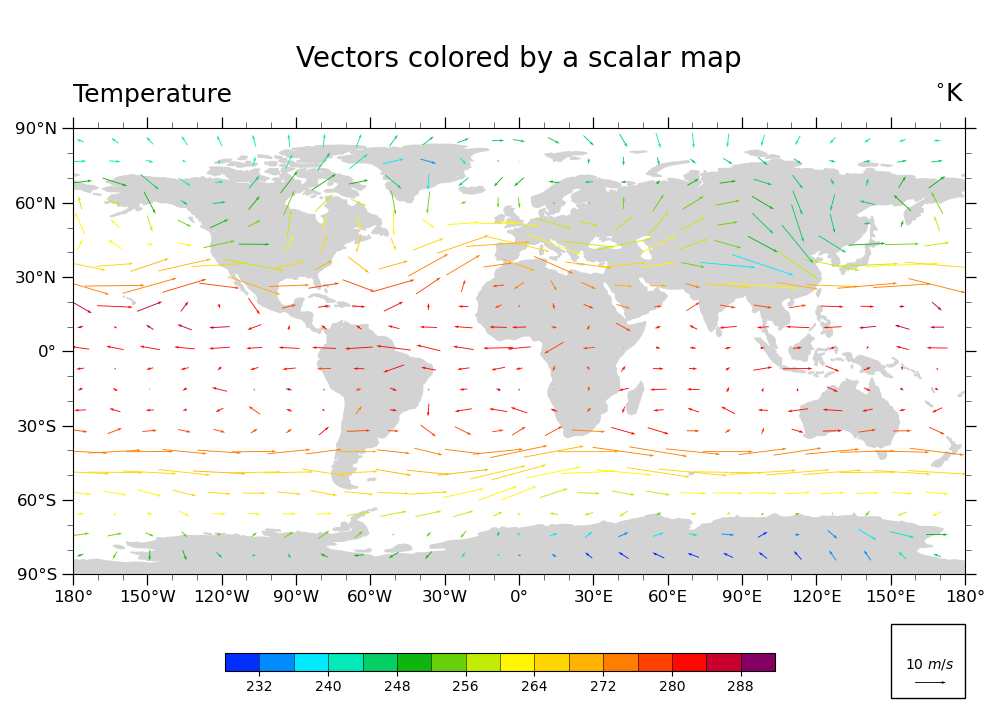# NCL_vector_4.py#

Plot U & V vectors globally, colored according to temperature

This script illustrates the following concepts:
• Coloring vectors based on temperature data

• Changing the scale of the vectors on the plot

See following URLs to see the reproduced NCL plot & script:

Import packages:

```import xarray as xr
from matplotlib import pyplot as plt
import cartopy
import cartopy.crs as ccrs
import cmaps

import geocat.datafiles as gdf
import geocat.viz as gv
```

```# Open a netCDF data file using xarray default engine and load the data into xarrays
file_in = xr.open_dataset(gdf.get("netcdf_files/83.nc"))

# Extract slices of lon and lat for first timestamp and 13th lev
ds = file_in.isel(time=0, lev=12, lon=slice(0, -1, 5), lat=slice(2, -1, 3))
```

Plot:

```# Because there is no equivalent to ``CurlyVector`` in ``geocat.viz`` yet,
# this plot does not look as identical as the NCL version.

# Generate figure (set its size (width, height) in inches)
fig = plt.figure(figsize=(10, 7.25))

# Generate axes using Cartopy projection
ax = plt.axes(projection=ccrs.PlateCarree())

# Import an NCL colormap and truncate it for a range and color levels
cmap = gv.truncate_colormap(cmaps.BlAqGrYeOrReVi200,
minval=0.03,
maxval=0.95,
n=16)

# Draw vector plot
# (there is no matplotlib equivalent to "CurlyVector" yet)
Q = plt.quiver(ds['lon'],
ds['lat'],
ds['U'].data,
ds['V'].data,
ds['T'].data,
cmap=cmap,
zorder=1,
pivot="middle",
width=0.001)
plt.clim(228, 292)

# Draw legend for vector plot
plt.Rectangle((150, -140),
30,
30,
facecolor='white',
edgecolor='black',
clip_on=False))
qk = ax.quiverkey(Q,
0.93,
0.06,
10,
r'10 \$m/s\$',
labelpos='N',
coordinates='figure',
color='black')

# Use geocat.viz.util convenience function to add minor and major tick lines

# Use geocat.viz.util convenience function to make plots look like NCL plots by using latitude, longitude tick labels

# Set major and minor ticks
plt.xticks(range(-180, 181, 30))
plt.yticks(range(-90, 91, 30))

# Use geocat.viz.util convenience function to add titles to left and right of the plot axis.
gv.set_titles_and_labels(ax,
maintitle="Vectors colored by a scalar map",
lefttitle="Temperature",
righttitle="\$^{\circ}\$K")

cax = plt.axes((0.225, 0.075, 0.55, 0.025))
cbar = fig.colorbar(Q,
ax=ax,
cax=cax,
orientation='horizontal',
ticks=range(232, 289, 8),
drawedges=True)

edgecolor='lightgray',
facecolor='lightgray',
zorder=0)

# Generate plot!
plt.tight_layout()
plt.show()
``````/home/docs/checkouts/readthedocs.org/user_builds/geocat-examples/checkouts/latest/Gallery/Vectors/NCL_vector_4.py:115: UserWarning: This figure includes Axes that are not compatible with tight_layout, so results might be incorrect.
plt.tight_layout()
```

Total running time of the script: ( 0 minutes 0.395 seconds)

Gallery generated by Sphinx-Gallery